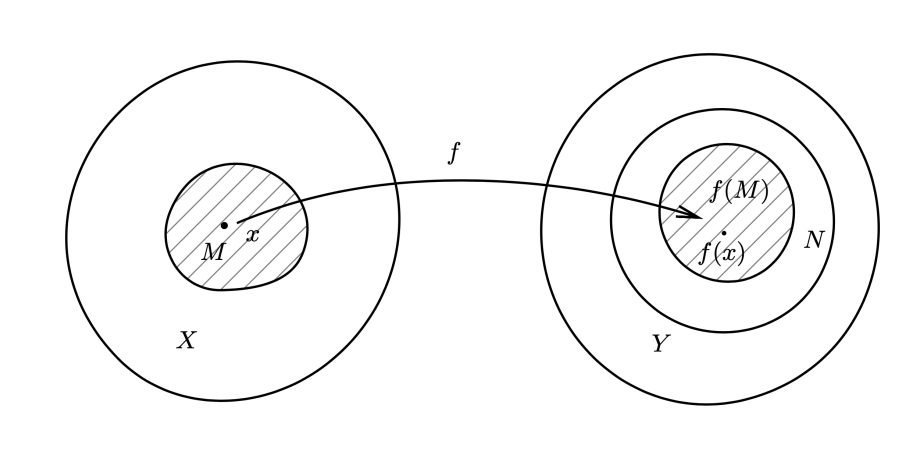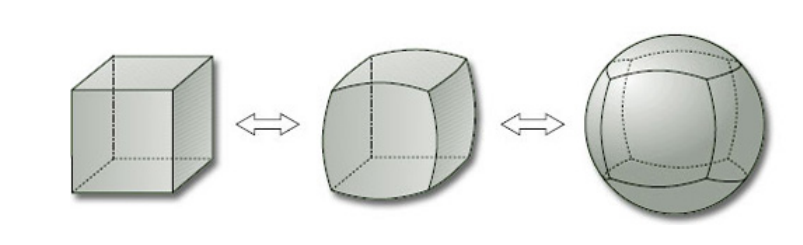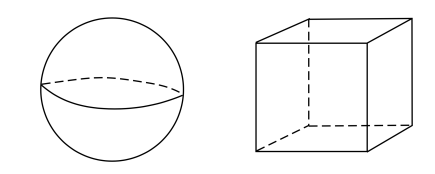## 拓扑空间连续映射

### 连续映射

$f$ 是由拓扑空间 $X$ 到另一个拓扑空间 $Y$ 的映射。$f$ 称为在点 $x \in X$ 是连续的，如果对于 $f(x)$ 的任意一个邻域 $N \subset Y$，都存在一个 $x$ 的一个邻域 $M \subset X$ 使得 $f(M) \subset N$• $f$ 称为在 $X$ 上连续，若其在 $X$ 的每一点都连续。
• 映射 $f : X \rightarrow Y$ 称为是连续的，若对于 $Y$ 中的每一个开集 $U$，集合 $f^{-1} (U)$$X$ 中是开集。

$X$ 的拓扑是离散拓扑，则所有映射 $f:X\rightarrow Y$ 都是连续的。
$Y$ 的拓扑是平凡拓扑，则所以的 $f$ 也是连续的。

### 同胚

$f : X \rightarrow Y$ 是一个双射（$f^{-1}$ 存在）， 若 $f$$f^{-1}$ 都连续，则称 $f$ 为同胚。• 不撕裂：这种操作可以当作映射
• 不粘合：所成的映射是单射
• 连续的：邻近的点变换后仍保持邻近（连续映射）
• 所谓的 拓扑不变量 是指拓扑空间的在同胚下被保持的某些性质。 例如：连通性，紧致性等等。拓扑不变量在数学和物理上是非常重要的。
• 反过来，拓扑不变量是否一定要求在同胚变换下不变？答案是否定的。一些拓扑不变量只要求映射为同伦，并不一定要求同胚。

## 相对拓扑和乘积拓扑

$A \subset X$$X$的拓扑为 $\mathscr{U}$。子集 $A$ 的相对拓扑定义为：

$\mathscr{U}_A = \{A \cap U|U \in \mathscr{U} \}$

$S^{n-1}$$\mathbb{R}^n$ 的子集，$\mathbb{R}^n$ 的通常拓扑诱导出 $S^{n-1}$ 上的相对拓扑使其成为拓扑空间。

$(X,\mathscr{U} )$$(Y,\mathscr{V} )$ 是两个拓扑空间。直积 $X \times Y$ 上可以赋予拓扑 $\{(U,V )|U \in \mathscr{U} ,\mathscr{V} \in V \}$ 这个拓扑称为 $X \times Y$ 上的乘积拓扑。

$\mathbb{R}^n = \mathbb{R}\times \cdots \times \mathbb{R}$ 中可以认定“开球”：

$\sum_{i=1}^{n}(x^i-a^i)^2 < b^2$## 拓扑结构和代数结构

### 拓扑群

$(X,\cdot)$ 是一个群。若 $X$ 上存在一个拓扑使得所有的乘法和求逆是连续的，即如下两个映射：

\begin{aligned} &X \times X \rightarrow X, (x,y) \mapsto x \cdot y = xy ,\\ &X \rightarrow X, x \mapsto x ^{-1}\\ \end{aligned}

$\mathrm{GL}(n,\mathbb{R}) := \{A \in M(n,\mathbb{R})|\det A\neq 0 \}$

### 拓扑矢量空间

$\mathbb{K}$ 上的线性空间称为是拓扑线性空间，若它可以赋予一个拓扑使得矢量加法，标量积运算都是连续的，即映射：

\begin{aligned} &X \times X \rightarrow X , (x,y) \mapsto x + y\\ &\mathbb{K} \times X \rightarrow X , (\lambda,x) \mapsto \lambda x\\ \end{aligned}

$\mathbb{R}^n$ 赋以通常拓扑成为一个拓扑矢量空间，称为 欧几里德空间。有时记为 $\mathbb{E}^n$$\mathbb{R}^n$ 在加法下形成一个阿贝尔群，因此在赋以通常拓扑后，它也形成一个阿贝尔连续群。